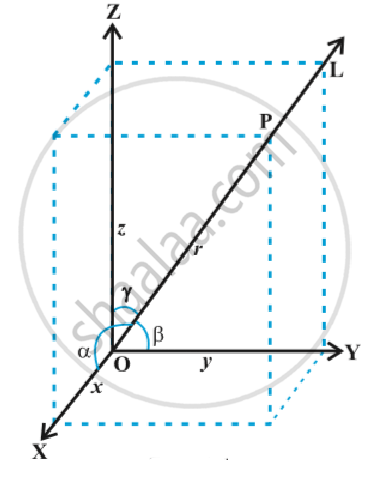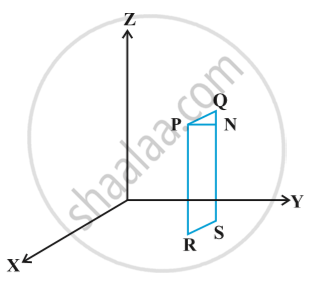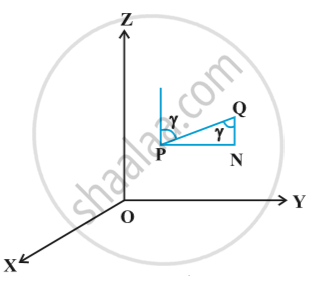Direction Cosines and Direction Ratios of a Line

notes

A directed line L passing through the origin makes angles α, β and γ with x, y and z-axes, respectively, called direction angles, then cosine of these angles, namely, cos α, cos β and cos γ are called direction cosines of the directed line L.
If we reverse the direction of L, then the direction angles are replaced by their supplements, i.e., π - α  , π-β  and π-γ  . Thus, the signs of the direction cosines are reversed.in explain in  following fig.A given line in space can be extended in two opposite directions and so it has two sets of direction cosines. In order to have a unique set of direction cosines for a given line in space, we must take the given line as a directed line. These unique direction cosines are denoted by l, m and n.

Remark: If the given line in space does not pass through the origin, then, in order to find its direction cosines, we draw a line through the origin and parallel to the given line. Now take one of the directed lines from the origin and find its direction cosines as two parallel line have same set of direction cosines.

Any three numbers which are proportional to the direction cosines of a line are called the direction ratios of the line. If l, m, n are direction cosines and a, b, c are direction ratios of a line, then a = λl,  b=λm and  c = λn, for any nonzero λ ∈ R.

Let a, b, c be direction ratios of a line and let l, m and n be the direction cosines (d.c’s) of the line. Then
l/a = m/b = n/c = k   ("say")   k being a constant.
Therefore l = ak , m=bk , n= ck        ...(1)
But l^2 + m^2 + n^2 = 1
Therefore k^2(a^2 + b^2 + c^2) = 1
Or  k = +- 1/ sqrt(a^2 + b^2 + c^2)
Hence, from (1), the d.c.’s of the line are

l = +- a/sqrt(a^2 + b^2 + c^2)  ,

m = +- b/sqrt(a^2 + b^2 + c^2) ,

n = +- c/sqrt(a^2 + b^2 + c^2)

where, depending on the desired sign of k, either a positive or a negative sign is to be taken for l, m and n.
For any line, if a, b, c are direction ratios of a line, then ka, kb, kc ;  k ≠ 0 is also a set of direction ratios. So, any two sets of direction ratios of a line are also proportional. Also, for any line there are infinitely many sets of direction ratios.

Direction cosines of a line passing through two points :
The direction cosines of a line passing through the given points P(x_1, y_1, z_1) and Q(x_2, y_2, z_2) as following fig.Let l, m, n be the direction cosines of the line PQ and let it makes angles α, β and  γ with the x, y and z-axis, respectively.
Draw perpendiculars from P and Q to XY-plane to meet at R and S. Draw a perpendicular from P to QS to meet at N. Now, in right angle triangle PNQ, ∠PQN= γ in following fig.Therefore, cos γ = (NQ)/(PQ) = (z_2 - z_1)/(PQ)

Similarly cos α = (x_2 - x_1)/(PQ) and cos β =(y_2 - y_1)/(PQ)
Hence, the direction cosines of the line segment joining the points P(x_1, y_1, z_1) and Q(x_2, y_2, z_2)  are

(x_2 - x_1)/(PQ)  , (y_2 - y_1)/(PQ)  , (z_2 - z_1)/(PQ)

where PQ = sqrt ((x_2 - x_1)^2 + (y_2 - y_1)^2 + (z_2 - z_1)^2)

If you would like to contribute notes or other learning material, please submit them using the button below.

Video Tutorials

We have provided more than 1 series of video tutorials for some topics to help you get a better understanding of the topic.

Series 1

Series 2

Series 3

Shaalaa.com

Three Dimensional Geometry Part 1- Direction Ratios [00:33:19]
S
0%